# Linear Equations and Inequalities: Business Math Lesson Plans Chapter Exam

Exam Instructions:

Choose your answers to the questions and click 'Next' to see the next set of questions. You can skip questions if you would like and come back to them later with the yellow "Go To First Skipped Question" button. When you have completed the practice exam, a green submit button will appear. Click it to see your results. Good luck!

### Page 1

#### Question 1 1. This graph below shows the growth of an oak tree. The x axis represents days, and the y axis represents inches. Interpolate what happens on day 3.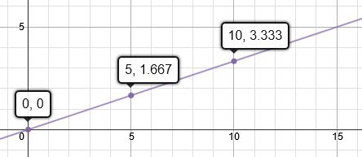### Page 2

#### Question 9 9. Solve the following system of equations.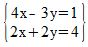#### Question 10 10. Which linear equation is shown below?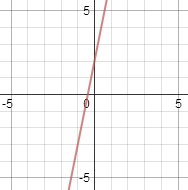### Page 3

#### Question 11 11. Which variable or variables need to be eliminated from the third equation in order to solve via the Gaussian elimination method?#### Question 15 15. What can you do to eliminate the x in the second equation?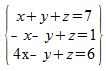### Page 4

#### Question 17 17. What is the equation of the graph below?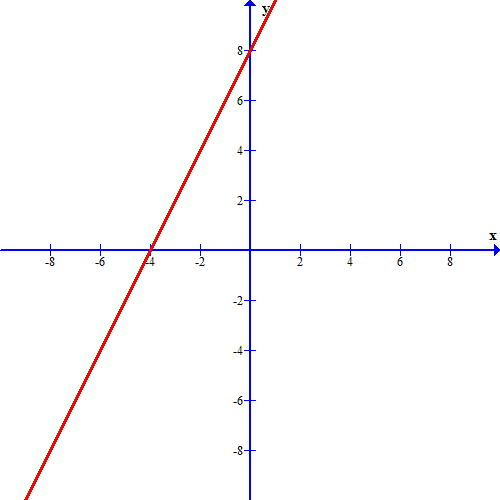#### Question 18 18. Which of the following points is an extrapolation of the graph below?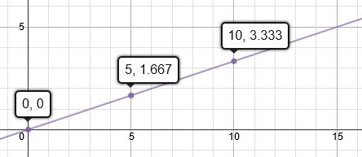### Page 5

#### Question 22 22. From the graph below, how many data points were collected?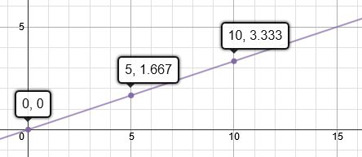#### Question 23 23. Which variable in which equation needs to be eliminated in order to solve this system using Gaussian elimination?#### Question 24 24. Which of these equations represents a line that is perpendicular to the line in the graph below?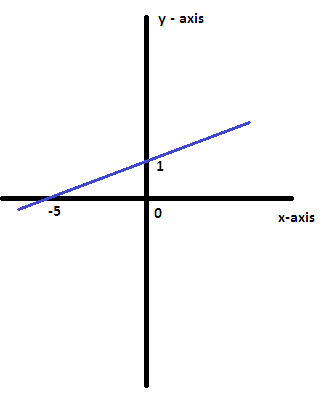### Page 6

#### Linear Equations and Inequalities: Business Math Lesson Plans Chapter Exam Instructions

Choose your answers to the questions and click 'Next' to see the next set of questions. You can skip questions if you would like and come back to them later with the yellow "Go To First Skipped Question" button. When you have completed the practice exam, a green submit button will appear. Click it to see your results. Good luck!

Support